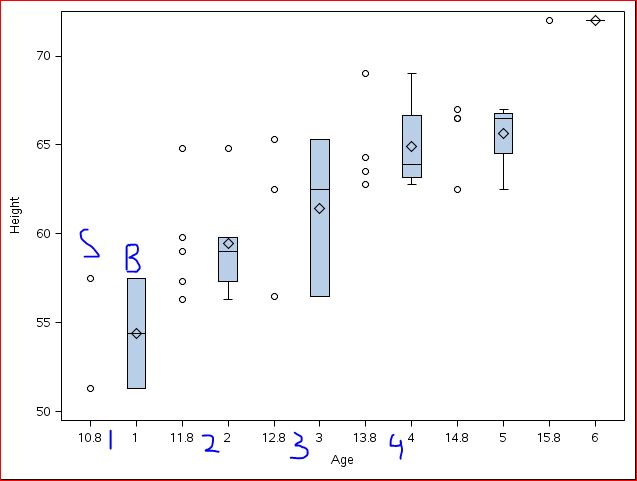## x axis labels? in overlapping scatter plot and boxplot

Dear Experts,

This is a questions with regard to the x axis labels i want to put in the graph where there is scatter plot and box plot jiterred.

i have written the following code

data class;

set sashelp.class;

age2=age-0.2;

run;

proc format fmtlib ;

value ns 10-11='1'

11<-12='2'

12<-13='3'

13<-14='4'

14<-15='5'

15<-16='6'

;

run;

proc template ;

define statgraph scatterbox ;

begingraph ;

layout overlay / xaxisopts=(linearopts=(VIEWMIN=10 tickvaluesequence=(start=10 end=16 increment=1)));

boxplot x=age y=height / xaxis=x ;

scatterplot x=age2 y=height / xaxis=x ;

endlayout;

endgraph;

end ;

proc sgrender data=class template=scatterbox ;

format age  ns.;

run ;

the graph generated is as belowThe scatter plot and box plot are aligned side by side. However the issue i have is with the x axis label, i should assign the label as shown in blue and that label should be between the scatter plot and box plot as labelled.

Thanks,

Jag

Thanks,
Jag
1 ACCEPTED SOLUTION

Accepted SolutionsJay54
Meteorite | Level 14

## Re: x axis labels? in overlapping scatter plot and boxplot

Please ALWAYS include the release of SAS you are running.  Thanks for including the full program with data.

If you are running SAS 9.3 or later, you can user DiscreteOffset to place a scatter and a box side by side using GTL.  You can use the same variable for X or Category, The variable can be either discrete or a numeric column with a format.

2 REPLIES 2Jay54
Meteorite | Level 14

## Re: x axis labels? in overlapping scatter plot and boxplot

Please ALWAYS include the release of SAS you are running.  Thanks for including the full program with data.

If you are running SAS 9.3 or later, you can user DiscreteOffset to place a scatter and a box side by side using GTL.  You can use the same variable for X or Category, The variable can be either discrete or a numeric column with a format.

## Re: x axis labels? in overlapping scatter plot and boxplot

Thank you Sanjay! it worked.

i am working on sas 9.3.

Regards,

Jag

Thanks,
Jag
Discussion stats
• 2 replies
• 1726 views
• 0 likes
• 2 in conversation